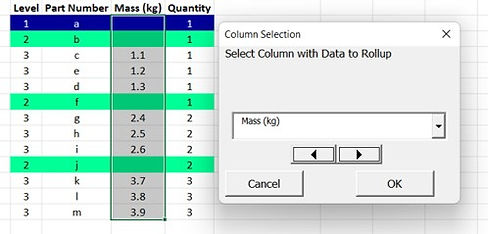top of page

# Rollup Equations

Anchor 2

## Consider the following table that has structure, in which the parent rows have been highlighted for clarity using the using the Highlight tool.  The table has part numbers, a Quantity column, and a 'Mass' column. Suppose you would like to know how much the entire system weighs.  You can not simply add up all of the cells in the 'Mass' column as that would neglect both the structure and the quantities.## Step 1:  Click on the RollupEqns function.## Step 2:  Select the column of the parameter that you would like to rollup by using the arrow buttons or the dropdown.  In this case, 'Mass' in column 'G', has been selected.## Step 3:  The 'Rollup Equation Builder' form appears and allows the user to select column inwhich to write the equations.  In this case it is the column immediately to the right of the table, Column "I".  The 'Quantity' column is detected automatically, but can be manually selected as well.## Step 4:  Click on 'Insert Equations'.  The equations are automatically written in the selected column, which in this case was the column immediately to the right of the table.  The title is automatically written, noting the source data column.  If the 'Quantity' column is missing, the function will merely write the equations with respect to the structure, assuming a quantity of one (1) for each item.## ​Anchor 1

Anchor 3

Option2

## There are some situations where this is necessary, for instance in manufacturing.  The total labor content is not merely the content required to build sub-assemblies, but to assembly the sub-assemblies into the top level item.  The labor to do so can be considered to be at the top level.Option3

## This option allows users to write rollup equations considering "Make/Buy" decisions.  A BOM may have numerous components that are purchased in an assembly (PIA).  The cost contribution of these parts might not be known.  But if the value of the assembly is known then adding up the children is not necessary and only the value of the parent is needed.  Conversely, if the assembly will be made internally, then the cost of each constituent part will be necessary to build the proper equations to determine the total cost of the end item.## In this case the second line item is more mature than the least mature child.  As such this value is used in lieu of the sum of the children.  As you can see this can make a difference in the result.## This function checks for the presence of a parent value.  If one is found it is assumed to be more mature than the sum of the children.## Exceptions and Highlights:

Exceptions## Also, note in the form above that both the 'Add Exception Comments', and the 'Highlight Exceptions' have been checked.  The rollup value with the exception has been highlighted and has a comment of explanation.bottom of page